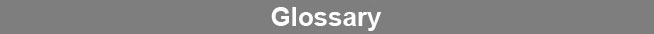Select Page### Weighting Equation

The weighting equation describes the average variance of Y response points as a function of the mean of those responses. The weighting equation is computed from a regression of the means and variances of the Y responses from the weighting assays. The weighting equation is used to weight data reduction regressions.www.brendan.com